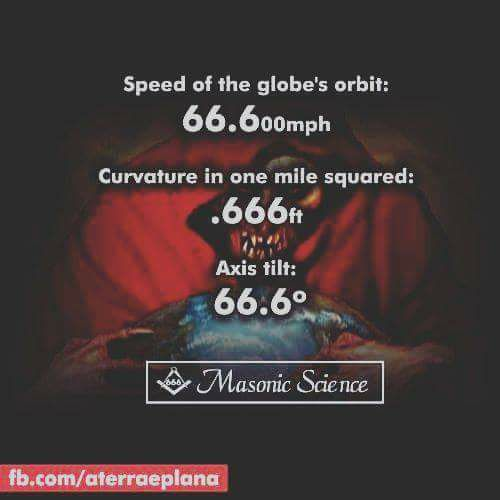666 ... globe earth math

Here is wisdom. Let him that hath understanding count the number of the beast: for it is the number of a man; and his number is Six hundred threescore and six.- Revelation 13:18

The following is some numerology taken from spherical earth figures that is interesting.

"Speed of the globe’s orbit: 66.600mph"
"Curvature in one mile squared: 666ft"
"The earth is on a 66.6 tilt."
"Earth's axis of rotation and its plane of orbit around the Sun: 66.6 degree"
"The polar circles are located near the poles of the Earth, at 66.6° N and S latitude."
"Distance to the nearest galaxy is 11.7 million light-years.." 11+7=18 (6+6+6=18)
"The nearest solar system (star Gliese 876) is 15.3 light years away..." 15+3=18 (6+6+6=18)
"There are 1800 confirmed new worlds outside of our Solar System." (6+6+6=18)
"Pluto has 18% the mass of our Moon." (6+6+6=18)
"Pluto's moon 'Charon' is nearly 1/8 the mass of Pluto." (6+6+6=18)
"The newest planet discovered 2016 (Proxima b) is 4.22 light-years away". 4-22=18 (6+6+6=18)
"The Solar System is traveling at an average speed of 828,000 km/h" 8+2+8=18 (6+6+6=18)
"The Sun was formed from the solar nebula about 4.59 billion years ago." 4+5+9=18 (6+6+6=18)
"The Earth's orbital speed around the Sun: 108,000 km/h" 10+8=18 (6+6+6=18)
"The total journey time from Earth to Mars takes between 150-300 days" 15+3=18 (6+6+6=18)
"Neptune's equatorial clouds take 18 hours to make one rotation." (6+6+6=18)
"The Moon was formed 4.6 billion years ago around some 30–50 million years after the formation of the solar system" 4+6+3+5= 18 (6+6+6=18)
"Pluto's distance changes from 7.3 billion kilometers to 4.4 billion kilometers." 7+3+4+4=18 (6+6+6=18)
"Milky Way is moving towards Centaurus at a speed of between 150 and 300 miles/sec" 15+3=18 (6+6+6=18)